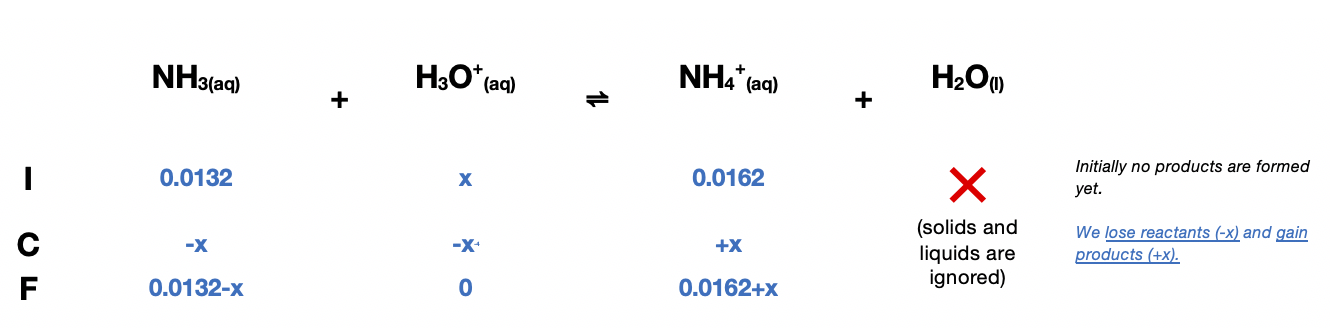# Problem: A 120.0 -mL buffer solution is 0.110 M in NH3 and 0.135 M in NH4Br. What mass of HCl can this buffer neutralize before the pH falls below 9.00?

###### FREE Expert Solution

Determine the moles of the weak acid and conjugate base:

0.0132 mol

0.0162 mol

Construct an ICF Chart:$\overline{){\mathbf{pH}}{\mathbf{=}}{{\mathbf{pK}}}_{{\mathbf{a}}}{\mathbf{+}}{\mathbf{log}}\mathbf{\left(}\frac{{\mathbf{NH}}_{\mathbf{3}}}{{{\mathbf{NH}}_{\mathbf{4}}}^{\mathbf{+}}}\mathbf{\right)}}$

${\mathbf{pK}}_{\mathbf{a}}\mathbf{=}\mathbf{14}\mathbf{-}{\mathbf{pK}}_{\mathbf{b}}$

${\mathbf{pK}}_{\mathbf{a}}\mathbf{=}\mathbf{14}\mathbf{-}\mathbf{4}\mathbf{.}\mathbf{75}\mathbf{=}$9.25

${\mathbf{9}}{\mathbf{=}}{\mathbf{9}}{\mathbf{.}}{\mathbf{25}}{\mathbf{+}}{\mathbf{log}}\mathbf{\left(}\frac{{\mathbf{NH}}_{\mathbf{3}}}{{{\mathbf{NH}}_{\mathbf{4}}}^{\mathbf{+}}}\mathbf{\right)}\phantom{\rule{0ex}{0ex}}\phantom{\rule{0ex}{0ex}}{\mathbf{log}}\mathbf{\left(}\frac{{\mathbf{NH}}_{\mathbf{3}}}{{{\mathbf{NH}}_{\mathbf{4}}}^{\mathbf{+}}}\mathbf{\right)}{\mathbf{=}}{\mathbf{9}}{\mathbf{-}}{\mathbf{9}}{\mathbf{.}}{\mathbf{25}}\phantom{\rule{0ex}{0ex}}\phantom{\rule{0ex}{0ex}}{\mathbf{log}}\mathbf{\left(}\frac{{\mathbf{NH}}_{\mathbf{3}}}{{{\mathbf{NH}}_{\mathbf{4}}}^{\mathbf{+}}}\mathbf{\right)}{\mathbf{=}}{\mathbf{-}}{\mathbf{0}}{\mathbf{.}}{\mathbf{25}}$

$\mathbf{\left(}\frac{{\mathbf{NH}}_{\mathbf{3}}}{{{\mathbf{NH}}_{\mathbf{4}}}^{\mathbf{+}}}\mathbf{\right)}{\mathbf{=}}{{\mathbf{10}}}^{\mathbf{-}\mathbf{0}\mathbf{.}\mathbf{25}}\phantom{\rule{0ex}{0ex}}\phantom{\rule{0ex}{0ex}}\mathbf{\left(}\frac{{\mathbf{NH}}_{\mathbf{3}}}{{{\mathbf{NH}}_{\mathbf{4}}}^{\mathbf{+}}}\mathbf{\right)}{\mathbf{=}}{\mathbf{0}}{\mathbf{.}}{\mathbf{5623}}\phantom{\rule{0ex}{0ex}}\phantom{\rule{0ex}{0ex}}\mathbf{\left(}\frac{\mathbf{0}\mathbf{.}\mathbf{0132}\mathbf{-}\mathbf{x}}{\mathbf{0}\mathbf{.}\mathbf{0162}\mathbf{+}\mathbf{x}}\mathbf{\right)}{\mathbf{=}}{\mathbf{0}}{\mathbf{.}}{\mathbf{5623}}$

$\mathbf{\left(}\frac{\mathbf{0}\mathbf{.}\mathbf{0132}\mathbf{-}\mathbf{x}}{\mathbf{0}\mathbf{.}\mathbf{0162}\mathbf{+}\mathbf{x}}\mathbf{\right)}{\mathbf{=}}{\mathbf{0}}{\mathbf{.}}{\mathbf{5623}}\phantom{\rule{0ex}{0ex}}\phantom{\rule{0ex}{0ex}}{\mathbf{0}}{\mathbf{.}}{\mathbf{5623}}\left(0.0162+x\right){\mathbf{=}}{\mathbf{0}}{\mathbf{.}}{\mathbf{0132}}{\mathbf{-}}{\mathbf{x}}\phantom{\rule{0ex}{0ex}}\phantom{\rule{0ex}{0ex}}{\mathbf{9}}{\mathbf{.}}{\mathbf{10926}}{\mathbf{×}}{{\mathbf{10}}}^{\mathbf{-}\mathbf{3}}{\mathbf{+}}{\mathbf{0}}{\mathbf{.}}{\mathbf{5623}}\left(x\right){\mathbf{=}}{\mathbf{0}}{\mathbf{.}}{\mathbf{0132}}{\mathbf{-}}{\mathbf{x}}\phantom{\rule{0ex}{0ex}}\phantom{\rule{0ex}{0ex}}{\mathbf{0}}{\mathbf{.}}{\mathbf{5623}}\left(x\right)+x={\mathbf{0}}{\mathbf{.}}{\mathbf{0132}}{\mathbf{-}}{\mathbf{9}}{\mathbf{.}}{\mathbf{10926}}{\mathbf{×}}{{\mathbf{10}}}^{\mathbf{-}\mathbf{3}}\phantom{\rule{0ex}{0ex}}\phantom{\rule{0ex}{0ex}}\frac{\overline{)\mathbf{1}\mathbf{.}\mathbf{5623}}\mathbf{x}}{\overline{)\mathbf{1}\mathbf{.}\mathbf{5623}}}{\mathbf{=}}\frac{\mathbf{4}\mathbf{.}\mathbf{09074}\mathbf{×}{\mathbf{10}}^{\mathbf{-}\mathbf{3}}}{\mathbf{1}\mathbf{.}\mathbf{5623}}$

88% (472 ratings)###### Problem Details

A 120.0 -mL buffer solution is 0.110 M in NH3 and 0.135 M in NH4Br. What mass of HCl can this buffer neutralize before the pH falls below 9.00?

Frequently Asked Questions

What scientific concept do you need to know in order to solve this problem?

Our tutors have indicated that to solve this problem you will need to apply the Buffer concept. You can view video lessons to learn Buffer. Or if you need more Buffer practice, you can also practice Buffer practice problems.

What professor is this problem relevant for?

Based on our data, we think this problem is relevant for Professor Rex's class at UCF.